Courses

# Second Law Of Thermodynamics MCQ Level – 2 (Part - 1)

## 10 Questions MCQ Test Basic Physics for IIT JAM | Second Law Of Thermodynamics MCQ Level – 2 (Part - 1)

Description
This mock test of Second Law Of Thermodynamics MCQ Level – 2 (Part - 1) for Physics helps you for every Physics entrance exam. This contains 10 Multiple Choice Questions for Physics Second Law Of Thermodynamics MCQ Level – 2 (Part - 1) (mcq) to study with solutions a complete question bank. The solved questions answers in this Second Law Of Thermodynamics MCQ Level – 2 (Part - 1) quiz give you a good mix of easy questions and tough questions. Physics students definitely take this Second Law Of Thermodynamics MCQ Level – 2 (Part - 1) exercise for a better result in the exam. You can find other Second Law Of Thermodynamics MCQ Level – 2 (Part - 1) extra questions, long questions & short questions for Physics on EduRev as well by searching above.
QUESTION: 1

### The property of a working substance which increases or decreases as the heat is supplied or removed in a reversible manner is known as. Select one:

Solution: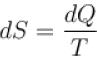Hence, entropy increases or decreases as heat is supplied or removed in reversible manner.

QUESTION: 2

### An ideal gas has temperature T1 at the initial state i shown in the p-V diagram here. The gas has a higher temperature T2 at final states a and b, which it can reach along the path shown. The entropy change along the path to state a is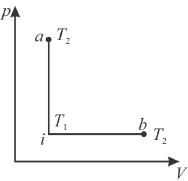Select one:

Solution:

From i to a and i to b, change in temperature is same, hence dU remains same for both the processes. But i to a is isochoric process, hence work done is zero.
⇒ heat gained in process a is smaller, hence the entropy change along the pathto state a is smaller than b.
The correct answer is: smaller than b

QUESTION: 3

### The T-S diagram of two cycles for the operation of an engine are shown in figure below.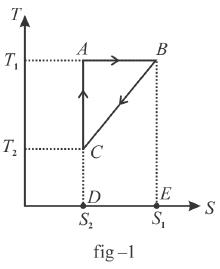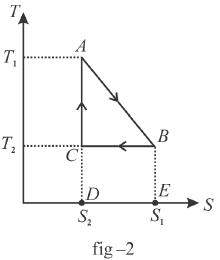The numerical values of the parameters T1 , T2 , S1 and S2 in the two figures are the same. Which cycle has greater efficiency? Select one:

Solution:

Efficiency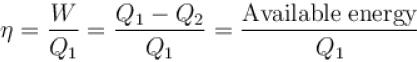Available energy per cycle is = Q1 – Q2
= Area of curve ABC
Q1 = Total area of curve (ABCD)
⇒ Area of curve is same for both figures, but total area is more for figure 1.
⇒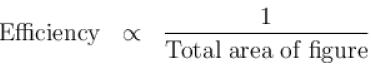Hence, second figure, has greater efficiency

QUESTION: 4

The difference in entropy between a state of volume Vi and a state of volume of Vf (temperature and number of molecules remaining constant) is equal to.
Select one:

Solution:

dS =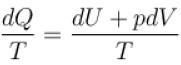for constant temperature, dU = 0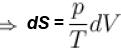and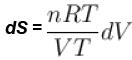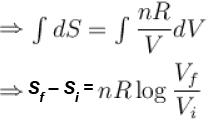The correct answer is: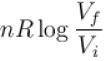QUESTION: 5

The efficiency of the Carnot cycle may be increased by.
Select one:

Solution: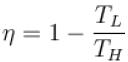if TL is reduced, η can be increased.
The correct answer is: decreasing the lowest temperature

QUESTION: 6

Kelvin-Plank’s law deals with
Select one:

Solution:

Kelvin-Plank statement of the second law of thermodynamics states that it is impossible to devise a cyclically operating device, the sole effect of which is to absorb energy in the form of heat from a single thermal reservoir and to deliver an equivalent amount of work.
The correct answer is: conversion of heat into work

QUESTION: 7

In a reversible process, entropy of the system.
Select one:

Solution:

For all reversible processes and constitutes part of the second law of thermodynamics, the entropy of the universe remains constant in a reversible process.

QUESTION: 8

1gm of perfect gas at volume VA , pressure pA and temperature TA changes from state A to state B when volume is VB, pressure is pB and temperature TB. the change in entropy is.
Select one:

Solution:

Change in entropy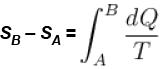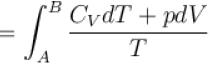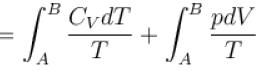pV = RT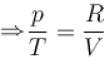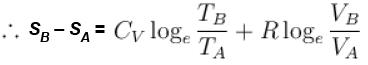The correct answer is: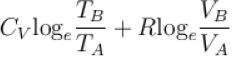QUESTION: 9

Change in entropy depends
Select one:

Solution:

Entropy is a measure of the number of microscopic configurations that a thermodynamic system can have when in a state as specified by some macroscopic variable.
The correct answer is: on the thermodynamic state

QUESTION: 10

In an irreversible process, the entropy of the universe.
Select one:

Solution:

When dealing with a system that is not isolated from its surroundings, remember that the increase in entropy described in the second law is that of the system and its surroundings. When a system and its surrounding interact in an irreversible process, the increase in entropy of one is greater than the decrease in entropy of the other. Hence, we conclude that the change in entropy of the universe must be greater than zero for an irreversible process and equal to zero for a reversible process.